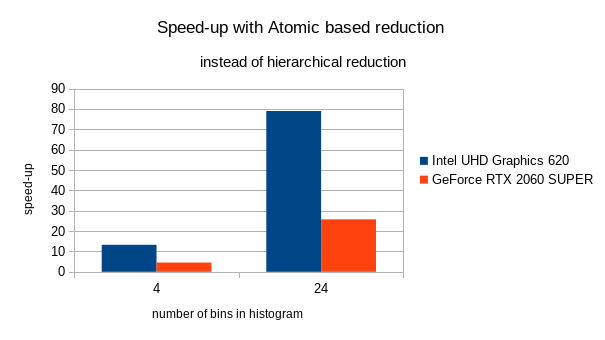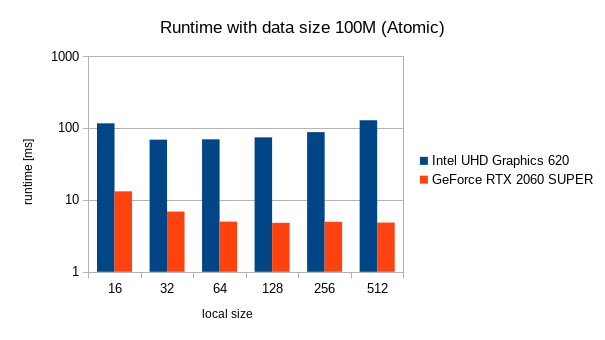This post aims to explain two approaches to histogram calculation on GPU using OpenCL and to compare the performance. We want to calculate the histogram with n bins. The aim is to count the number of records in each interval. The query is something like

SELECT count(*) FROM data GROUP BY intervals


I implemented two algorithms that solve this problem:

• hierarchical reduction
• reduction based on the atomic operation

#### Hierarchical reduction

The hierarchical reduction algorithm reduces the data using a binomial tree scheme. The OpenCL code can be

__kernel void reduce(__global const float *x, __global float *sums, __local float *localSums, float a, float b, int n)
{
uint lid = get_local_id(0);
uint gid = get_global_id(0);
uint group_size = get_local_size(0);

if(x[gid] >= a && x[gid] < b && gid < n){ /* is the record in interval [a, b) */
localSums[lid] = 1.0;
}
else{
localSums[lid] = 0.0;
}

for (uint stride=group_size/2; stride>0; stride /= 2){
barrier(CLK_LOCAL_MEM_FENCE);
if(lid < stride){
localSums[lid] += localSums[lid + stride];
}
}
if(lid == 0){
sums[get_group_id(0)] = localSums[lid];
}
}


As you can see, every iteration of for loop reduces the data in local memory to half. The buffer sums contains the partial counts associated with computation groups and the final result must be computed in the host. This code counts only the records in one interval so the code must be executed for every interval.

This is the classical solution that is fully atomic free. Literature:

#### Atomic based reduction

This algorithm uses the atomic operation for integers defined in OpenCL specification especially atomic_inc and atomic_add. The code is

#pragma OPENCL EXTENSION cl_khr_local_int32_base_atomics : enable
#pragma OPENCL EXTENSION cl_khr_global_int32_base_atomics : enable

__kernel void reduce_count(__global const float *x, __global int *sums, __local int *localSums, float lo, float hi, int numBins, int n)
{
uint lid = get_local_id(0);
uint gid = get_global_id(0);
uint group_size = get_local_size(0);
uint group_id = get_group_id(0);

if (lid < numBins){
localSums[lid] = 0;
}
barrier(CLK_LOCAL_MEM_FENCE);

float step = (hi - lo)/numBins;   /* compute interval step */

if(gid < n){
int index = (int) ((x[gid] - lo) / step);   /* compure the interval number */
if (index == numBins){
index -= 1;
}
atomic_inc(&localSums[index]);   /* increase the local count for interval 'index' */
}
barrier(CLK_LOCAL_MEM_FENCE);

if (lid < numBins){
}
}


This code generates the histogram directly so the kernel is performed only once. First, the data are reduced in local memory and then in global memory. The buffer sums contains the final histogram. The performance of this solution highly depends on the speed of the atomic operation.

#### Performance

The tests were performed on two graphic cards: Intel UHD Graphics 620 and GeForce RTX 2060 SUPER.

In the following graph, there are the results of performance tests.The atomic based reduction is much faster than hierarchical reduction on selected graphics cards. The histogram generation for 100M data size takes less than 5 ms on GeForce RTX 2060 SUPER.